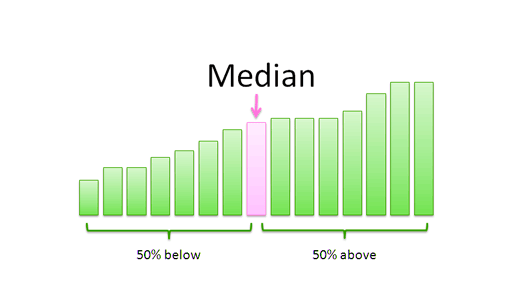# Median

Median, in statistics, is the middle value of the given list of data, when arranged in an order. The arrangement of data or observations can be done either in ascending order or descending order

Example: The median of 2,3,4 is 3.

In Maths, the median is also a type of average, which is used to find the center value. Therefore, it is also called measure of central tendency

Apart from the median, the other two central tendencies are mean and mode. Mean is the ratio of sum of all observations and total number of observations. Mode is the value in the given data-set, repeated most of the time.

In geometry, a median is also defined as the center point of a polygon. For example, the median of a triangle is the line segment joining the vertex of triangle to the center of the opposite sides. Therefore, a median bisects the sides of triangle.

## Median in Statistics

The median of a set of data is the middlemost number or center value in the set. The median is also the number that is halfway into the set.

To find the median, the data should be arranged, first, in order of least to greatest or greatest to the least value. A median is a number that is separated by the higher half of a data sample, a population or a probability distribution, from the lower half. The median is different for different types of distribution.For example, the median of 3, 3, 5, 9, 11 is 5. If there is an even number of observations, then there is no single middle value; the median is then usually defined to be the mean of the two middle values: so the median of 3, 5, 7, 9 is (5+7)/2 = 6.

## Median Formula

The formula to calculate the median of the finite number of data set is given here. Median formula is different for even and odd numbers of observations. Therefore, it is necessary to recognise first if we have odd number of values or even number of values in a given data set.

The formula to calculate the median of the data set is given as follow.

### Odd Number of Observations

If the total number of observation given is odd, then the formula to calculate the median is:

Median = {(n+1)/2}thterm

where n is the number of observations

### Even Number of Observations

If the total number of observation is even, then the median formula is:

Median  = [(n/2)th term + {(n/2)+1}th]/2

where n is the number of observations

## How to Calculate the Median?

To find the median, place all the numbers in the ascending order and find the middle.

Example 1:

Find the Median of 14, 63 and 55

solution:

Put them in ascending order: 14, 55, 63

The middle number is 55, so the median is 55.

Example 2:

Find the median of the following:

4, 17, 77, 25, 22, 23, 92, 82, 40, 24, 14, 12, 67, 23, 29

Solution:

When we put those numbers in the order we have:

4, 12, 14, 17, 22, 23, 23, 24, 25, 29, 40, 67, 77, 82, 92,

There are fifteen numbers. Our middle is the eighth number:

The median value of this set of numbers is 24.

Example 3:

Rahul’s family drove through 7 states on summer vacation. The prices of Gasoline differ from state to state. Calculate the median of gasoline cost.

1.79, 1.61, 2.09, 1.84, 1.96, 2.11, 1.75

Solution:

By organizing the data from smallest to greatest, we get:

1.61, 1.75, 1.79, 1.84 , 1.96, 2.09, 2.11

Hence, the median of the gasoline cost is 1.84. There are three states with greater gasoline costs and 3 with smaller prices.

## Mean Median Mode

Let us see an example here to find mean, median and mode of the observations.

For example, 2,6,9,12,12 is the given set of data

Thus,

Median = Middle Value = 9

Mean = Sum of observations/Number of observations = (2+7+9+12+12)/5 = 41/5 = 8.2

Mode = Value repeated most number of times = 12

For more Maths-related articles, register with BYJU’S – The Learning App and download the app to learn with ease.

## Frequently Asked Questions – FAQs

### What is the Median? Give Example.

A median is the center value of a given list of observations when arranged in an order.
For example, a list of observations is 33, 55, 77, 22, 11.
Arranging in ascending order, we get:
11,22,33,55,77
Hence, the median is 33.

### What is the median of two numbers?

If the number of given set of observations is 2, then we have to apply the formula of median for even number of observations, i.e.
Median = [(n/2)th term + {(n/2)+1}th term]/2
Example: Median of 15 and 20 is: [(15)+(20)]/2 = 35/2 = 17.5

### What is the median of 10 number of observations?

The median of 10 numbers of observations is: (5th term + 6th term)/2

### What is the median of odd numbers of observations?

The formula to find median of odd number of observations is:
Median = [(n+1)th term]/2
Where n is the number of observations.

### What is the difference between mean and median?

Median is defined as the center value of ordered list of values.
Mean is the ratio of sum of list of values and number of values, order of values does not matter.